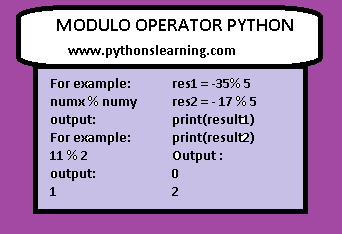# modulo operator in python 3.9

Welcome everyone, Today we will see Modulo Operator python,Modulo Operator python for negative number,How does a modulo operator work?,What is the use of modulo operator?,How do you find modulo?,What is the symbol of modulus operator?
so let’s start:

Modulo operator is show by the percent sign (%).
The syntax for Modulo Operator python is :

numx % numy

output:

For example

11 % 2

output:

1

If any case the divisor result is equal to zero then output show ZeroDivisionError:

11 % 0

output:

ZeroDivisionError: modulo by zero.

The modulo operator also work on floating numbers as arguments:
for example:

4.8 % 1.2

Copy

0.0

Remember % character represents the interpolation operator.(formatting strings)
Examples
modulo operator is used for different purpose like One of the most common use is to check whether a number is even or odd.
If a given number divided by 2 and has no remainder, then this number is even number. Otherwise it is odd.
EXAMPLE 01) Check for even or odd number:

numx = 15
` `

if (numx % 5) == 0:
` `

print(num, “is even no.”)
` `

else:
` `

print(num, “is odd no.”)
` `

output:
` `

15 is even no.

If you run the code above, 15 % 5 leaves a remainder of 0 and the code inside the else statement is executed:
15 is even no.
EXAMPLE 02) Check for prime number:

` `

if numb < 1:
` `

return False
` `

for i in range(2, numb):
` `

if (numb % i) == 0:
` `

return False
` `

else:
` `

return True

In this example first, we have to checking if the number, num is a positive number or negative(it’s required positive number). Next step is checking whether the number is divisible by another number in the range from 2 to number without any reminder. If none of the conditions are satisfy, the result is prime number.

## Modulo Operator python for negative number:

Most complex mathematics task is taking modulo of a negative number, which is done behind the program of Python.
(x+y)mod z = [(x mod z)+(y mod z)]mod z
To apply this math to given statement as –

-3 % 7 = ( -1*7 + 4 ) % 7 = 4

This was done so that the (-1*7)%7 will give the answer as 4
Let’s see more examples for better understanding.
Example #1 :
By using this mathematics, we can see able to perform and understand the negative modulo.

11 % 0
` `

output:
` `

ZeroDivisionError: modulo by zero.

filter_none
edit
play_arrow
brightness_4
# Using negative modulo in python

res1 = -35% 5
` `

res2 = – 17 % 5
` `

print(result1)
` `

print(result2)
` `

Output :
` `

0
` `

2

Some FAQ about Modulo Operator python:

### 1.How does a modulo operator work?

The modulus operator  is a path or way to determine the remainder of a division in any mathematics problem. The modulo operations return the whole numbers instead of returning the result of the division

#### 2.What is the use of modulo operator?

The modulo operations return the whole numbers instead of returning the result of the division. Modulo is indicated with a percent sign in most of place. For example, “10 mod 5” or “10%5” returns 0, because 5 divide into 10 perfectly, without a remainder.
3.How do you find modulo?

How to calculate the modulo – an example
1. choosing the starting numbers
2. Then Choose the divisor.
3. Divide one number by divisor
4. and multiply the divisor by the quotient.
5. Subtract number
6. Finally we got modulo

4.What is the symbol of modulus operator?
mod, or indicated  by the symbol %
Tags: Modulo Operator python,Modulo Operator python for negative number,How does a modulo operator work?,What is the use of modulo operator?,How do you find modulo?,What is the symbol of modulus operator?
If you want to learn data visualization then click on it.
BEST OF LUCK!!!

### sachin PagarI am Mr. Sachin pagar Embedded system engineer, the founder of Pythonslearning, a Passionate Educational Blogger and Author, who love to share the informative content on educational resources.

### sachin Pagar

I am Mr. Sachin pagar Embedded system engineer, the founder of Pythonslearning, a Passionate Educational Blogger and Author, who love to share the informative content on educational resources.